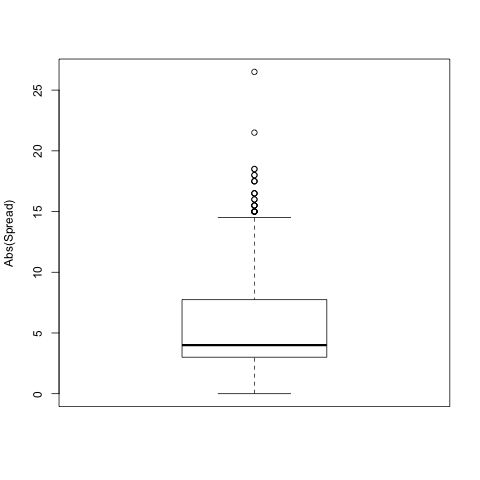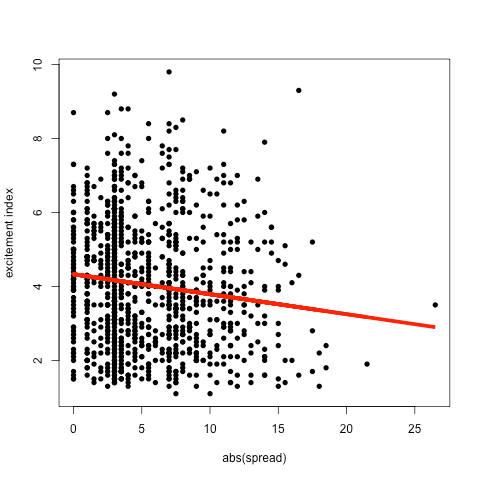### Introduction

Recently I tweeted the following question: “Does the spread of an NFL game predict how exciting the game will be?”  If closer games are considered more exciting (I think most people will agree with this), then games with smaller spreads should be, on average, more exciting than games with larger spreads.

So how do we test this?  The first big question that we need to answer is this: how do we quantify “excitement” of a game?  Luckily, someone has already done this for us. Advance NFL Stats calculates a statistics they call the “excitement index” (EI).  It is defined as follows on their website:

Excitement Index (EI) – The measure of how exciting a game is. EI measures the total movement of the Win Probability (WP) line during a game. The more that WP fluctuates, the more dramatic, uncertain, and exciting a game is.

I think this is a great definition of excitement, and whether or not you totally agree that this is or is not the best way to measure this, it is certainly a great place to start.

So to begin with I collected the EIs and spreads for all regular season games from 2010 through 2013 for a total of 1024 games.

### Summaries of the data

Looking at a summary of the absolute value of the spread over these 1024 games we find that the average spread is 5.44 points with a median of 4.  The inner quartile range of spreads in from 3 to 7.625 and the minimum spread over this time was 0 with a maximum of 26.5(!).The average EI is 4.036 with a median of 3.9.  The inner quartile range of the EI is from 2.7 through 5.2.  The least exciting game over this time period was measured to be 1.1 with the most exciting game at 9.8.

### Regression Model

Next, I fit a simple linear regression model with excitement as the response variable and absolute value of the spread as the predictor.  The fitted regression parameters are:

• Intercept: 4.33023 (p-value < 2e-16)

This means that for ever two point increase in spread, we lose, on average, about 1 point of excitement index.  In other words with a spread of 0, the average predicted excitement index (EI) of a game is 4.33023.  This is about the 60th percentile of all EIs from 2010-2013.  A spread of 3 yields a predicted excitement index of 4.168 with is about the 55th percentile of all excitement indices.  When the spread jumps to 7 points, the average excitement level is about 3.95 which is about the 51st percentile of EI.What can we expect about the most exciting game in a playoff weekend?  If we have four games all with spreads of 0, we can expect that the most exciting game will be about a 6 EI, which is about the 85th percentile of EI.  If all four games have a spread of 3 points we can expect the most exciting game to be about 5.9 EI, which is about the 84th percentile of EI, and if all 4 games have spreads of 7 we expect the most exciting game to be about 5.67 which is around the 81st percentile of games.  So what’s the difference between a 5.7 and a 6 for instance?  Here is an example of a 6 and here’s an example of a 5.7.  I guess the answer is not much.

### What can we expect in the Super Bowl?

The Super Bowl spread tonight is 2.5 points with Denver favored.  This gives us an expected excitement index of 4.19523, which is about the 55-th percentile of exciting games.  So we should expect the game tonight to be more exciting that than more than half of all regular season games played in the last four years.  And that is exciting.

Cheers.